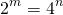# Exponents: Number Sense Activities

These should be approached without a calculator. A calculator might be used to check solution.

Number Sense Activity 1:

Compare the first five powers of 4 with the first ten powers of 2. Explain the pattern.  Choose various values ofandsuch that the following equation is true:Number Sense Activity 2:

Write three different equations, choosing values forandcarefully such that the equationis true. Check your equations with a calculator.

Number Sense Activity 3:

Which one of the following is true:Number Sense Activity 4:

Which is bigger;orNumber Sense Activity 5

Put the values in the correct place in the tableBall park Value Between 0 and 0.5 Between 0.5 and 1 More than 1 .

Number Sense Activity 6:

Which is bigger,or?